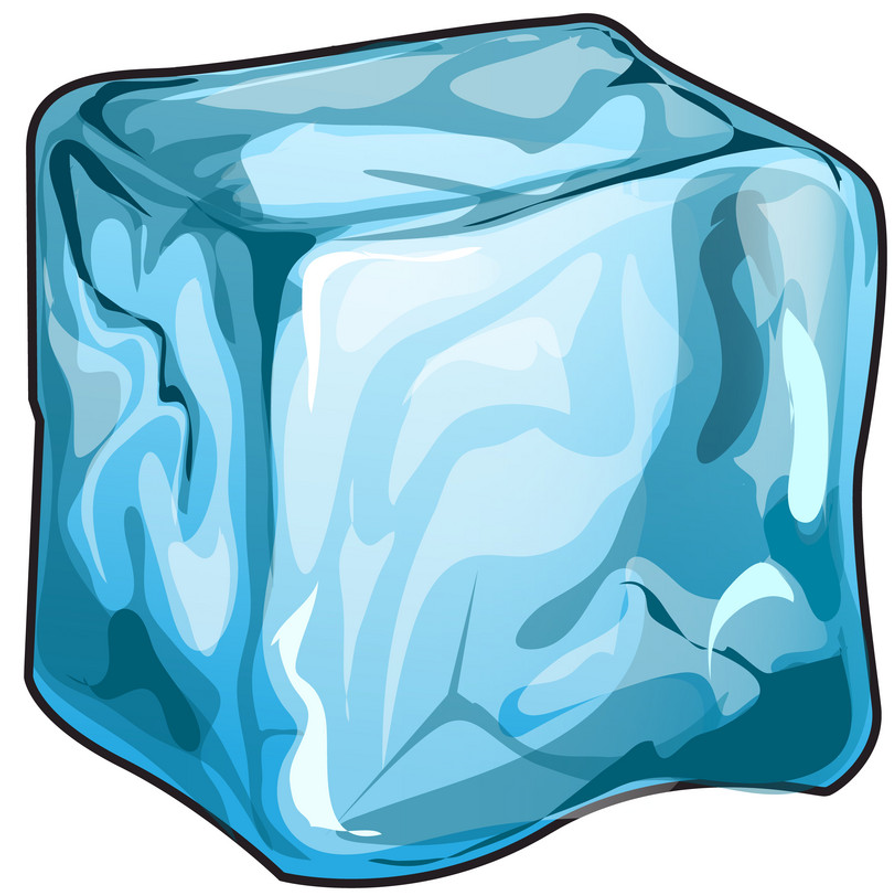# How many ice cubes?

Level 2How many ice cubes do you need to cool down $200g$ water from $25^{\circ}C$ to $5^{\circ}C$, if each cube is $6,4cm^3$ and $-5^{\circ}C$?(You drop them into the water)
Here are some constants:
$c_w=4200\frac{J}{kg\cdot K}$
$c_i=2100\frac{J}{kg\cdot K}$
$L_i=3.3\cdot 10^5\frac{J}{kg}$
$\rho _i=900\frac{kg}{m^3}$

If your answer is in $\overline{x.y}$ format, where $y<2$, then enter $x$, else if $y\geq2$ enter $x+1$.

A. P. Rimkevics-Physical task collection: 656(new)

×